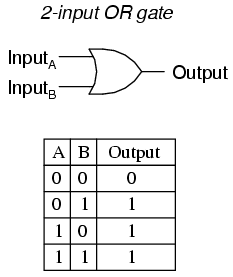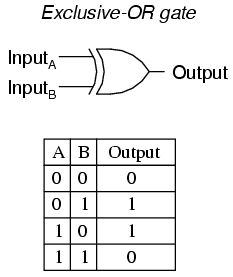# XOR Encryption¶

XOR encryption is one of the basic cipher's of Cryptography. It is based on the properties of the bitwise XOR gate. Before moving on to the real stuff, we will check out what Gates are and most importantly what a XOR Gate is.

## Logic Gates¶

Logic gates are the basic building blocks of any digital system. It is a mechanism to deal manipulate binary data. There are many basic Logic gates. But we will be discussing only which we need, they are

• AND gate
• OR gate
• XOR gate

### AND Gate¶

The AND gate is a logic circuit which gives a high output(1) only if all the inputs are high(1). A dot(.) is used to show the AND operation i.e. A.B or sometimes AB will suffice.### OR Gate¶

The OR gate is a logic circuit which gives a high output(1) if one or more of its inputs are high. A plus(+) is used to show the OR operation.### XOR gate¶

The Exclusive-OR or most commonly known as the XOR gate is a logic circuit which will give a low output(0) if either, but not both of its inputs are high(1). The $\oplus$ is used to represent the xor gate.Now that we have seen what Logic Gates are and what a xor gate is, lets move on to xor of two integers.

Lets take two integers 5 and 9. Now the binary equivalent of these two numbers is 00000101 and 00001001

 1 2 3 4  00000101 ⊕ 00001001 -------- 00001100 

The code for doing this in python is

>>> 5^9
12


The symbol ^ is used for xoring two numbers.

Now let us xor two two characters, let them be j and f. Now what happens here when you xor two characters is that, these are first converted into ascii form and then xored together, i.e. in python first we convert j and f into their respective ascii values, this can be done by the use of the ord() function

>>> ord('r')
114
>>> ord('j')
106


Now these values are xored togerther and converted back into their character form. This can be done by the use of chr(). The python code for xoring two characters is

>>> chr(ord('r') ^ ord('j'))
\x18

Here we get an unprintable character, which is normal in cryptography.

Important

The most important property of xor is that , if A ⊕ B = C then, B ⊕ C = A and also, C ⊕ A = B

Now let us move the encryption part.

## Single-Byte XOR Cipher¶

In this the key is going to be a single byte, i.e. the entire message is going to be xored with a single character (printable or non printable), which ranges in ascii value of 0 to 255.

To make it simpler let us take an example. Let us take a message "Hello" and xor it with the key which can be taken as "s" (Here we have used a printable character). What we do is that, we take the first character of the message i.e. H and xor it with the single-byte key i.e. s , the resultant will become the first character of the ciphertext, now take the second character message e and xor it with the key s the resultant second character of the ciphertext and so it goes on.

 1 2 3 4 5 6 7  H e l l o 01001000 01100101 01101100 01101100 01101111 ⊕ 01110011 01110011 01110011 01110011 01110011 s s s s s ------------------------------------------------ 00111011 00010110 00011111 00011111 00011100 ; \x16 \x1f \x1f \x1c 

Now we have the ciphertext. Now to get the message back repeat the same process with the ciphertext

 1 2 3 4 5 6 7  ; \x16 \x1f \x1f \x1c 00111011 00010110 00011111 00011111 00011100 ⊕ 01110011 01110011 01110011 01110011 01110011 s s s s s ------------------------------------------------ 01001000 01100101 01101100 01101100 01101111 H e l l o 

Usually you are not given the key, but you can easily decrypt the ciphertext. You could just try Bruteforcing it, what it means is that you could try all the possible keys. Since it is a single byte, it is easy because the the total possibilities is 256 characters.

## Repeated-Key XOR Cipher¶

In this, unlike Single-Byte Xor Cipher the key is made up of multiple bytes. Let us take an example to understand this, the message we are going to encrypt is Document with the key abc. The procedure is simple, first you xor the first character of the message, D with the first character of the key a, now the second character of the message o is xored with the second character of the key b, and the third character of the message c with the third character of the key c. Now that we are out of key characters, what should we do next? The answer is that we start over, the fourth character of the message is xored with the first character and so on, until the whole message is encrypted.

 1 2 3 4 5 6 7  D o c u m e n t 01000100 01101111 01100011 01110101 01101101 01100101 01101110 01110100 ⊕ 01100001 01100010 01100011 01100001 01100010 01100011 01100001 01100010 a b c a b c a b ------------------------------------------------------------------------------ 00100101 00001101 00000000 00010100 00001111 00000110 00001111 00010110 % \r \x00 \x14 \x0f \x06 \x0f \x16 

To get the real message back just repeat the same process with the ciphertext.

 1 2 3 4 5 6 7  % \r \x00 \x14 \x0f \x06 \x0f \x16 00100101 00001101 00000000 00010100 00001111 00000110 00001111 00010110 ⊕ 01100001 01100010 01100011 01100001 01100010 01100011 01100001 01100010 a b c a b c a b ------------------------------------------------------------------------------ 01000100 01101111 01100011 01110101 01101101 01100101 01101110 01110100 D o c u m e n t 

Let us look at the code in python to do this.

>>> pt = "Document"
>>> key = "abcabcab"
>>> "".join(chr(ord(i) ^ ord(j)) for i,j in zip(pt,key))
'%\r\x00\x14\x0f\x06\x0f\x16'
>>> ct = '%\r\x00\x14\x0f\x06\x0f\x16'
>>> "".join(chr(ord(i) ^ ord(j)) for i,j in zip(ct,key))
'Document'

Practice Challenges